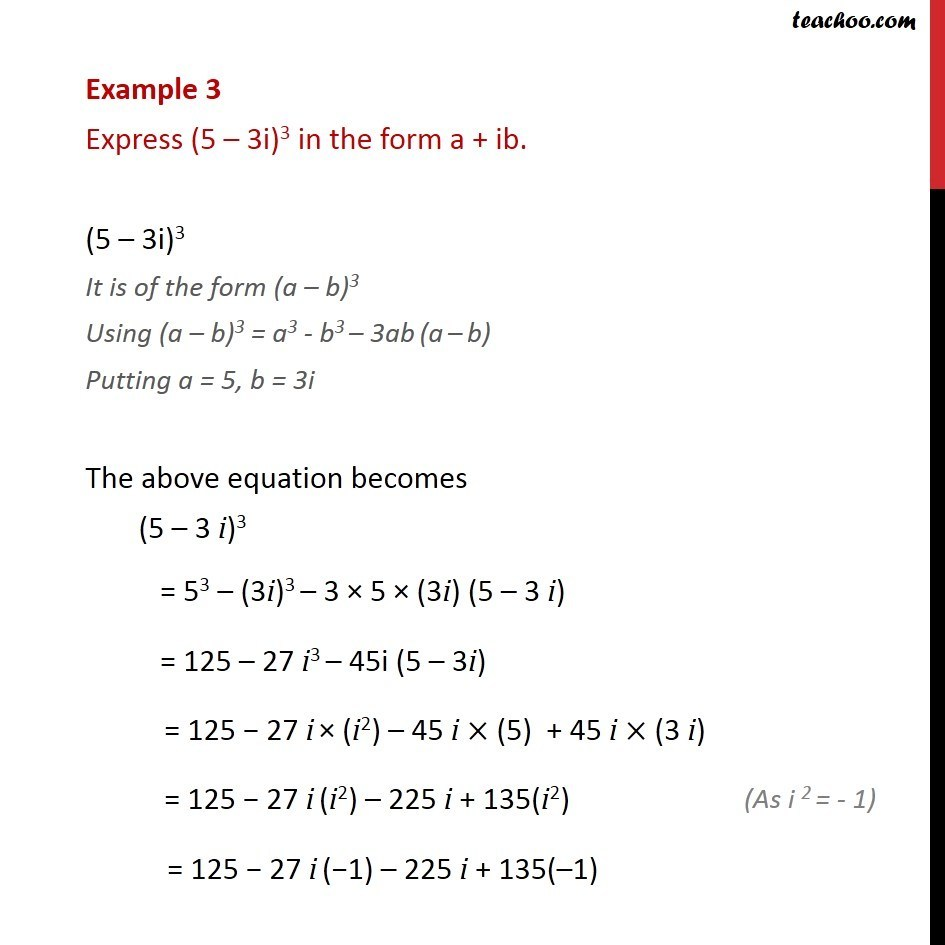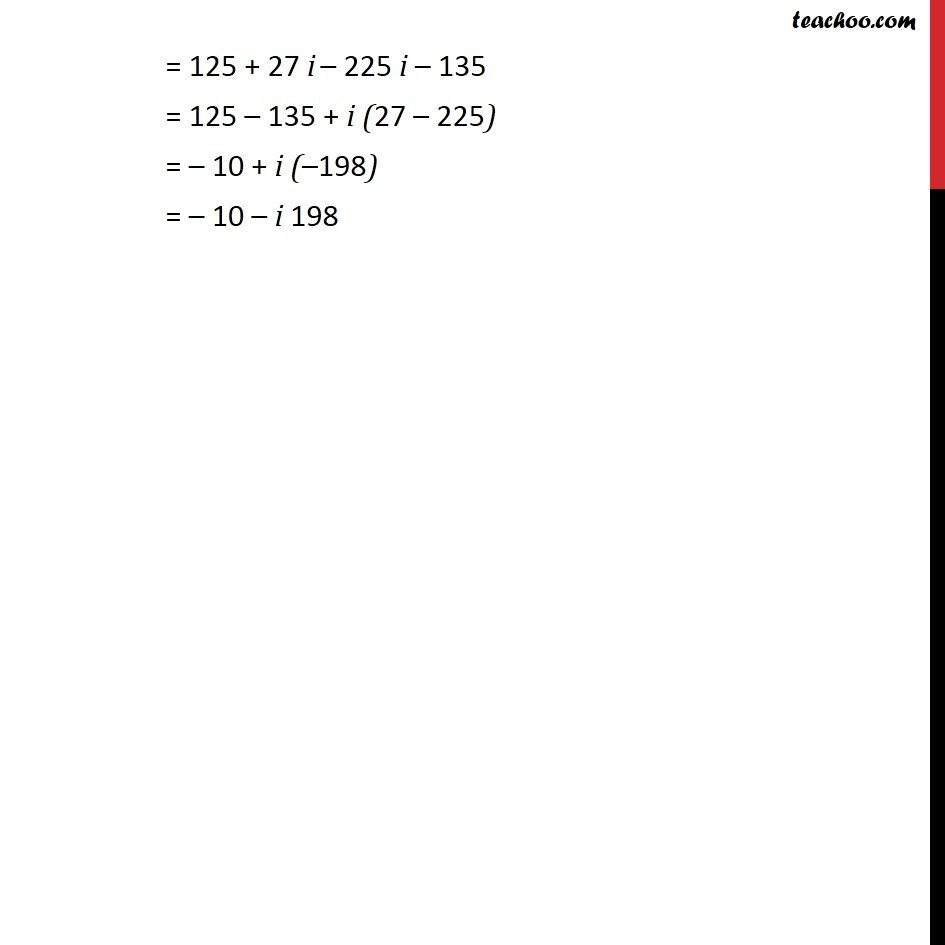Identities (square, cube of 2 complex numbers)

Chapter 5 Class 11 Complex Numbers (Term 1)
Concept wise### Transcript

Example 3 Express (5 – 3i)3 in the form a + ib. (5 – 3i)3 It is of the form (a – b)3 Using (a – b)3 = a3 - b3 – 3ab (a – b) Putting a = 5, b = 3i The above equation becomes (5 – 3 i)3 = 53 – (3i)3 – 3 × 5 × (3i) (5 – 3 i) = 125 – 27 i3 – 45i (5 – 3i) = 125 − 27 i × (i2) – 45 i × (5) + 45 i × (3 i) = 125 − 27 i (i2) – 225 i + 135(i2) = 125 − 27 i (−1) – 225 i + 135(–1) = 125 + 27 i – 225 i – 135 = 125 – 135 + i (27 – 225) = – 10 + i (–198) = – 10 – i 198